﻿ QSAR Study of a Serie of Benzimidazolylchalcone Derivatives by the Density Fonctional Theory (DFT) MethodPublications are Open
Access in this journal
Article Versions
Export Article
• Normal Style
• MLA Style
• APA Style
• Chicago Style
Research Article
Open Access Peer-reviewed

### QSAR Study of a Serie of Benzimidazolylchalcone Derivatives by the Density Fonctional Theory (DFT) Method

Doumadé ZON , Jean Stéphane N’DRI, Adéyolé TIMOTOU, Ahmont Landry Claude KABLAN, Mamadou Guy-Richard KONÉ, Mahama OUATTARA, Drissa SISSOUMA
Journal of Materials Physics and Chemistry. 2021, 9(1), 9-15. DOI: 10.12691/jmpc-9-1-2
Received March 08, 2021; Revised April 15, 2021; Accepted April 24, 2021

### Abstract

This QSAR study involved a series of benzimidazolylchalcone derivatives. It allowed us to obtain a model from the molecular descriptors and anthelminthical activity against Haemonchus contortus. The molecular descriptors were obtained by applying the methods of quantum chemistry at the B3LYP/6-31G (d) level. The statistical indicators of the model are: the coefficient of determination R2 equals 0.990, the standard deviation S equals 0.209, the Fischer coefficient F equals 153.055 and the cross-validation coefficient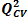equals 0.990. This model has good statistical performances. The quantum descriptors of dipole moment (μD) and electronic energy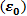are responsible of the anthelminthic activity of benzimidazolylchalcone derivatives. In addition, the dipole moment is the priority descriptor for the prediction of the antibacterial activity of the studied compounds. The Eriksson et al. acceptance criteria used for the test set is verified. The values of the pCL100theo/pCL100exp ratio of the theoretical and experimental activities for the test set aim towards the unit.

### 1. Introduction

The fight against infectious diseases remains a challenge of public health essential; this is explained by the high mortality and morbidity rate bound these diseases 1, 2. In addition the anti-infectious’s chemotherapies currently are inefectives because of drug-resistance’s phenomenon of infectious germs 3. In this context, the continuation of the development of new effective drugs turns out to be a vitally necessary. This QSAR study concerns a serie of nine (9) benzimidazolylchalcone derivatives including six (6) were of use to the training set and the three others to external validation set (Figure 1). These compounds were synthetized and tested by Zon Doumade et al. 4 for their anthelminthicals activities.

These compounds were obtained by Claisen-Schmidt reaction in basic solution. It is the condensation of 2-acétylbenzimidazole and derivatives with various benzaldehyds in ethanolic solution of sodium hydroxide. These molecules were tested against Haemonchus contortus. The general objective of this work is to make a descriptive and predictive study of the anthelminthic activity. The obtained model will allow to identify the best analogues called "lead" which will may fight more effectively against the parasitic staims.

• Figure 1. Molecular structures of benzimidazolylchalcone derivatives used for training and validation set of the QSAR model

### 2. Experimental Section

2.1. Materials and Methods of Calculation

The six molecules of the training set and the three other validation set molecules used in this study, have various anthelminthical concentrations ranging from 0.0005 to 12.03 μg/mL. This range of concentrations makes it possible to define a quantitative relationship between the anthelminthic activity and the theoretical descriptors. Biological data are generally expressed as the opposite of the log 10 base of activity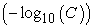in order to obtain higher mathematical values when the structures are biologically very efficient 5, 6. The anthelminthical activity is expressed by the anthelminthical potential pCL100which is defined by equation (1)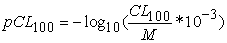(1)

Where M is the molecular weight (g/mol) and CL100 is the larvicid concentration, it gives the smallest concentration at which normal larval development is blocked (μg/mL).

2.2. Level of Calculation

The relation between the biological activity values of the molecules studied and their molecular structures was demonstrated by quantum chemistry calculations carried out using Gaussian software 7. DFT methods are generally known to generate a variety of molecular properties 8, 9, 10, 11, 12, 13, 14, 15 in QSAR studies. These methods increase predictability, reduce computation time, and involve costs in the conception of new drugs 16, 17. The B3LYP / 6- 31G (d) theory level was used to determine the molecular descriptors. The modeling was done using the multilinear regression method implemented in Excel spreadsheets 18 and XLSTAT 19.

2.3. Quantum Descriptors

For the development of the QSAR model, some theoretical descriptors related to the conceptual DFT were determined such as the dipole moment (μD) and the electronic energy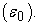The dipole moment (μD) indicates the stability of a molecule in the water. Thus, a high dipole moment will result in poor solubility in organic solvents and high solubility in water. The electronic energy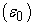represents the electronic contribution of all atoms in a given molecule. These descriptors are all determined from the optimized molecules. The calculation of the partial correlation coefficient between the pair of descriptors studied is less than 0.70 (aij <0.70); which means that these different descriptors are independent of each other 20.

2.4. Estimation of the Predictive Ability of a QSAR Model

The quality of a model is determined on the basis of various statistical analysis criteria including the coefficient of determination R2, the standard deviation S, the correlation coefficients of cross validation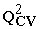and Fischer coefficient F. The statistical indicators R2, S and F relate to the adjustment of the calculated and experimental values. They describe the predictive capacity within the model limits and allow to estimate the precision of the values calculated on the training set 21, 22. As for the cross-validation coefficient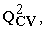it provides information on the predictive power of the model. This predictive power is called "internal" because it is calculated from the structures used to build this model. The determination’s coefficient R2 gives an evaluation of the dispersion of theoretical values around the experimental ones. The quality of the modeling is better when the points are close to the fitting line 23. The adjustment of the points to this line can be evaluated by the coefficient of determination.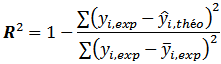(2)

Where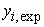: Experimental value of anthelminthical activity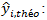Theoretical value of the anthelminthical activity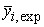: The mean value of the experimental values of the anthelminthical activity

The closer the value of R2 to 1, the more the theoretical and experimental values are correlated

Moreover, the variance σ2 is determined by the relation 3: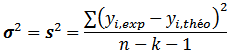(3)

Where k is the number of independent variables (descriptors), n is the number of molecules in the test or learning set and n-k-1 is the degree of freedom. The standard deviation S is another statistical indicator used. It evaluates the reliability and precision of a model: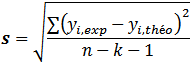(4)

The Fischer coefficient F is also used to measure the level of statistical significance of the model, ie the quality of the choice of the descriptors constituting the model. Its value must be greater than the critical value of the Fisher Snedecor table Fcr = 5.59 24.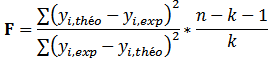(5)

The coefficient of determination of the cross-validationmakes it possible to evaluate the accuracy of the prediction on the test set and it is calculated by using the following equation: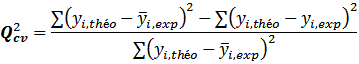(6)

The performance of a model, according to Eriksson et al. 24 is characterized by a value of> 0.5 for a satisfactory model and for the excellent modelis higher than 0.9. For a given training set, a model will be performing if the acceptance criterion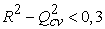is respected. Moreover, the predictive power of a model can be obtained from the value of the ratio pCL100 theo/ pCL100exp for the test set. The model is acceptable when the value of the ratio of theoretical and experimental activity tends towards unity.

2.5. Applicability Domain of a Model

The Applicability domain (AD) defines the area in which the activity of a compound can be confidently predicted. It therefore corresponds to the region of the chemical space including learning game compounds and similar compounds, close in the same space 26. There are several methods for determining of applicability domain of a model 27. We have used one of the most widely used leverage methods in this work. This method is based on the variation of the standardized residues of the dependent variable with the distance between the values of the descriptors and their mean, called leverage 28. A graphical representation of AD using standardized leverages and residues is referred to as the William graph 29. This graph allows detection of both outliers and influencing compounds in the model. The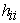leverage of compound i is defined by: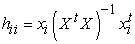(7)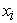is the line vector of the descriptors of compound i and X is the matrix built on the values of the descriptors of the model and the compounds of the learning game. The exponent t refers to the matrix or transposed vector.are the diagonal elements of an H matrix called hat matrix. H is the projection matrix of the experimental values of the explained variable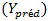in the space of the values of the predicted explained variablesuch as: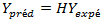(8)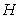is defined as (9) :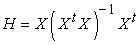(9)

The applicability domain is delimited by a threshold value of the leverage noted h*. In general, it is set to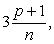where n is the number of learning game compounds, and p is the number of model descriptors 30, 31. For standardised residues, the two limit values generally used are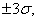σ being the standard deviation of the experimental values of the value to be explained 32: it is «the rule of the three sigmas» 33. If a compound has a lever that exceeds the critical value h* or a residue that is greater than +3σ or less than -3σ, then that compound is outside the applicability domain of the elaborated model 34, 35, 36.

### 3. Results and Discussion

3.1. Results

The training set of the six (6) molecules and the three (3) molecules of the test set are shown in Table 1. Thereafter, the values of the partial correlation coefficients aij of the descriptors are also presented in Table 2.

The partial correlation coefficients aij between the descriptor pairs (μD,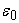) is less than 0.70 (aij <0.70). This demonstrates the independence of the descriptors used to develop the models.

3.2. QSAR Model Validation

The negative or positive sign of the descriptor’s coefficient of the model reflects the proportionality’s effect between the evolution of the anthelminthical activity and this descripor of the regression equation. Thus, the negative sign indicates that when the value of the descriptor is high, the biological activity decreases while the positive sign translates the opposite effect. The best QSAR models obtained for the anthelminthical activity against Haemonchus contortus as well as the statistical indicators are given below: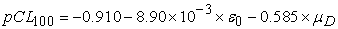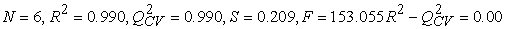The negative signs of the dipole moment and the electronic energy coefficients indicate that the anthelminthical activity will be improved for a low value of the dipole moment and the electronic energy. The Fisher test value of 153.055 is greater than the critical value (Fcr = 5.59). This shows that the error is less than what the model explains. The 0.990 value of the cross-validation coefficientwhich is greater than 0.9 translates an excellent model according to Erikson et al. This model is acceptable because the value of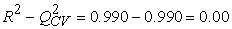is less than 0.3. The standard deviation (S = 0.209) expresses the small variation of the predicted values from the experimental mean.

External validation of the model was carried out with molecules (A, D, G). The values of the pCL100 theo/ pCL100exp ratio of the external validation set are shown in Table 3.

All values of the ratio pCL100 theo/pCL100exp tend to 1. This indicates the good correlation between the theoretical and experimental anthelminthic potentials of the studied benzimidazolylchalcones derivatives. This model is so acceptable for the prediction of the anthelminthical activity (Haemonchus contortus) of the series of benzimidazolylchalcones derivatives. The regression line between the experimental and theoretical anthelminthic activity of the training set (blue dots) and the test set (red dots) is illustrated in Figure 2.

• Figure 2. Graph between actual and predicted biological activity for training and test set of the model
• Figure 3. Contribution chart of differents descriptors in biological activity in the model
3.3. Analysis of the Contribution of Descriptors in the Model

The relative contribution of the descriptors in the prediction of the anthelminthical activity of the compounds is presented in Figure 3.

The dipole moment presents a wide contribution compared to electronic energy. Thus, the dipole moment proves to be the priority descriptor in the prediction of the anthelminthical activity (Haemonchus contortus) of the benzimidazolylchalcones derivatives studied.

3.4. Applicability Domain of Elaborated Model

The standardized residues, the values of the diagonal of the leverage matrix (hii) were identified. These different values are shown in Figure 4.

• Figure 4. William’s diagram of the model

Figure 4 shows that for the 6 learning game molecules and the two (2) model descriptors, the limit value of the leverages h* is 1.5. The maximum values for standardized residues are ±3 according to the “three sigma rule”. These values therefore delineate the applicability domain of the model as shown in the William diagram. In our work, we note that all leverages values for learning game molecules are lower than the lever threshold value h*(1.5). This translates a good chemical space of our mathematical model.

### 4. Conclusion

In this study, a QSAR model was developed on nine benzimidazolylchalcone derivatives to link anthelmintic activity against the parasite Haemonchus contortus with two quantum descriptors: dipolar moment (µD), and electronic energyMultiple linear regression (MLR) was used to quantify the relationship between these molecular descriptors and the inhibitory concentration potential (pCL100) of benzimidazolylchalcone derivatives. The statistical indicators of this model display the following values: R2 = 0.990, S = 0.209, F = 153.055. The validation techniques commonly used, namely the standard statistical indicators of the model, the external validation and the applicability domain, were used. This study revealed the existence of strong correlations between the calculated and experimental values of the anthelminthical potential. The obtained QSAR model allows us to predict the activity of the best analogues called "lead". This proposed model allows to identify the dipole moment as priority descriptor to improve the anthelminthic activity. Furthermore, the negative sign of the coefficient of dipole moment translates that the anthelminthic activity will be improved for a low value of this parameter. This study represents an orientation for the conception of new more active molecules against Haemonchus contortus. The performance of this model was verified by means of a test including three molecules. This study will play an important role in the understanding of the relation between the physico-chemical parameters of the structure and biological activity. By studying the QSAR model, we can select the suitable substituent and to conceive new compounds with an improved biological activity.

### ReferencesThis work is licensed under a Creative Commons Attribution 4.0 International License. To view a copy of this license, visit http://creativecommons.org/licenses/by/4.0/